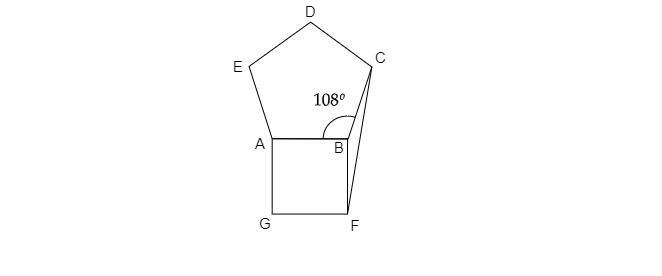# A regular pentagon $ABCDE$ and a square $ABFG$ are formed on opposite sides of $AB$. Find $\angle BCF$.

Given: A regular pentagon $ABCDE$ and a square $ABFG$ are formed on opposite sides of $AB$.

To do: To find $\angle BCF$.

Solution:As given, $ABCDE$ is a regular pentagon and $ABFG$ is a square.

So the sides of pentagon and square are equal.

Each angle of square $=90^o$

Each angle of regular pentagon$=108^o$

From the figure $\angle FBC=360-( \angle ABF+\angle ABC)$

$\Rightarrow \angle FBC = 360-( 90+108)$

$\Rightarrow \angle FBC=360-198=162^o$

In $\vartriangle BCF$,

$\because BF=FC$

$\therefore BFC=BCF$

$\Rightarrow \angle BCF+\angle BCF+162^o=180^o$

$\Rightarrow 2\angle BCF=180^o-162^o$

$\Rightarrow \angle BCF=\frac{18^o}{2}$

$\Rightarrow \angle BCF=9^o$

Updated on: 10-Oct-2022

40 Views